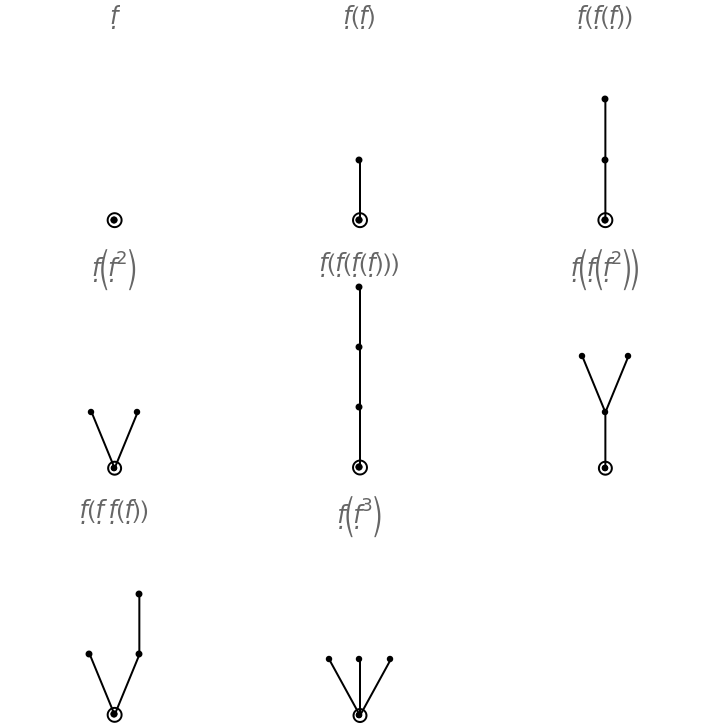#Function Repository Resource:

# ButcherTrees

Get a list of the trees for any Runge-Kutta method of a given order

Contributed by: Wolfram Research
 ResourceFunction["ButcherTrees"][p] gives a list, partitioned by order, of the trees for any Runge–Kutta method of order p.

## Details and Options

The trees are represented in a functional form in terms of the elementrary symbol f (\[FormalF]).

## Examples

### Basic Examples

Give the trees that are needed for any third-order method:

 In:=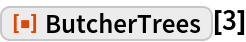Out=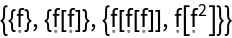### Properties and Relations

Check the trees are all valid syntax, using ResourceFunction["ButcherTreeQ"]:

 In:=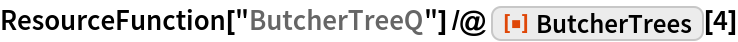Out=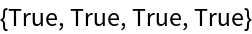ResourceFunction["ButcherPlot"] can be used to plot Butcher trees:

 In:=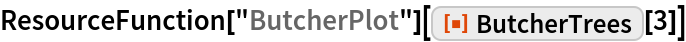Out=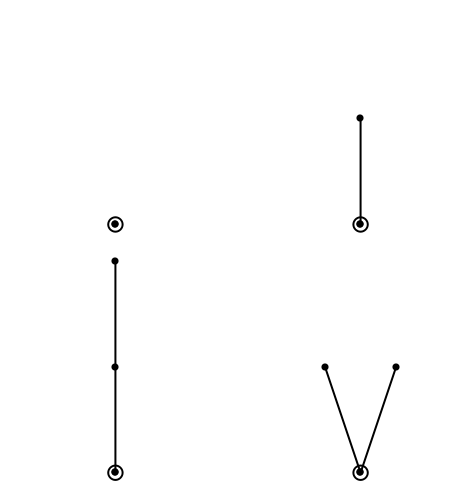### Neat Examples

Plot and label the trees through order 5:

 In:=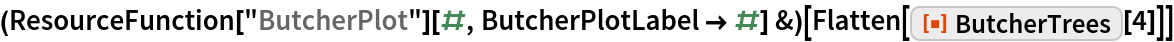Out=